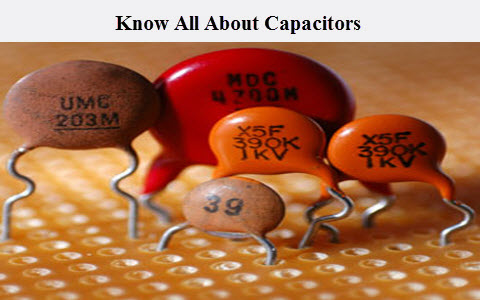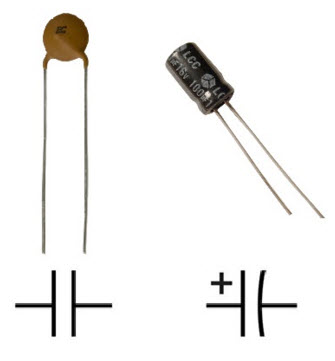# Know all About a Capacitor – Working of a Capacitor

Just like the various electrical and electronic components such as resistor, transistor, ICs, the capacitor is one of the most used components in electrical and electronic circuit design. Sometimes capacitor is referred as a condenser. It plays a vital role in various embedded applications. These components are obtainable at different ratings. It comprises of two metal plates that are parted by a dielectric or a non conducting substance. There are various kinds of capacitors are available in the market, but the difference between these capacitors are normally made with the dielectric material used in the plates. Some capacitors look like tubes, some capacitors are designed with ceramic materials and dipped into an epoxy resin to cover them. This article gives an overview of what is a capacitor, capacitor working and construction of a capacitor.## What is a Capacitor?

The capacitor is a two terminal electrical conductor and that is separated by an insulator. These terminals store electric energy when they connected to a power source. One terminal stores positive energy and the other terminal stores negative charge. Charging and discharging of the capacitor can be defined as, when electrical energy is added to a capacitor is called charging whereas releasing the energy from a capacitor is called as discharging.The capacitance can be defined as, it is the amount of electric energy stored in the capacitor at 1 volt and it is measured in units of Farad denoted by F. The capacitor separates current in DC (direct current) circuits and short circuit in AC (alternating current) circuits. The capacitance of a capacitor can be increased in three ways such as

• Increase the plate size
• Arrange the plates nearer together
• Make the dielectric good if possible

Capacitors include dielectrics made from all kinds of materials. In transistor radios, the changing is carried out by a variable capacitor that has air in between its plates. In most electrical and electronic circuits, these components are wrapped components by dielectrics made of ceramic materials like glass, mica, plastics or paper soaked in oil.

## Construction of a Capacitor

The simplest form of a capacitor is “ parallel plate capacitor” and its construction can be done by two metal plates that are placed parallel to each other at some distance.

If a voltage source is connected across a capacitor where the +Ve (positive terminal) is connected to the positive terminal of a capacitor and negative terminal is connected to –Ve (negative terminal) of the capacitor. Then, the energy which is stored in the capacitor is directly proportional to the applied voltage.

Q= CV

Where ’C’ is a proportionality constant, which is familiar as the capacitance of the capacitor. The unit capacitance of the capacitor is the Farad. According to the equation Q=CV, 1 F = coulomb/ volt. From the above equation, we can conclude that capacitance depends on voltage and charge, but this is not true. The capacitance of the capacitor mainly depends on the sizes of the plates and dielectric among two plates.
C= ε A/ d

The capacitance of the capacitor mainly depends upon the surface area of each plate, the distance between two plates and the permitivity of the material between the two plates.

### Basic Circuits of a Capacitor

Basic circuits of a capacitors mainly includes capacitors connected in series and capacitors connected in parallel.

#### Capacitors Connected in Series

When the two capacitors C1 and C2 are connected in series are shown in the circuit below.

When the capacitors C1 and C2 are connected in series, then the voltage from the voltage source is divided into V1 and V2 across the capacitors. The overall charge will be the charge of the entire capacitance

Voltage V= V1 + V2

The flow of current in any series circuit is throughout same

Thus, the total capacitance of the above circuit is C total= Q/V

We know that V= V1+ V2

= Q/ (V1 + V2)

The total capacitance of capacitors in series C1, C2

1/ CTotal = 1/ C1 + 1/ C2

Therefore, when a circuit having “n” number of capacitors connected in series

1/ CTotal = 1/ C1 + 1/ C2+…………..+1/Cn

#### Capacitors Connected in Parallel

When the two capacitors C1 and C2 are connected in parallel are shown in the circuit below.

When the capacitors C1 and C2 are connected in parallel, then the voltage from the voltage source will be the same across the capacitors. The charge in the first capacitor C1 will be Q1 and the charge in the second capacitor C2 will be Q2. Therefore, the equation can be written as

C1 = Q1/V and C2= Q2/ V

Therefore, when a circuit having “n” number of capacitors connected in parallel

C Total = C1 + C2+………….. +Cn

### Capacitance Measurement

The capacitance can be defined as, the amount of electrical energy stored in a capacitor used in a circuit (The unit of the capacitance is the Farad). The following 3 steps discuss about how to measure capacitance when the voltage and charge of a capacitor are known.

#### Find Out the Carrying Charge in the Capacitor

The charge is frequently problematic to measure directly. Because the unit of the ampere, the current is defined as 1 coulomb/Sec, if the current and the amount of time the current applied is known, it is conceivable to figure out the charge. You can simply get the charge in coulomb by multiplying the amperes in the time in seconds

For instance, if the capacitor has a current of 20 Amp applied to it for 5 Sec, the charge is 100 coulombs or 20 times 5.

#### Voltage Measurement

The voltage measurement can be done by using a voltmeter or multimeter by setting the voltage.

#### Divide the Electric Charge by the Voltage

A capacitor carrying 100 coulomb charge and a potential difference of a capacitor is 10volts then the capacitance would be 100 divided by 10.

Don’t Miss: Capacitor Color Code Calculation

Thus, this is all about what is a capacitor and capacitor working.We hope that you have got a better understanding of this concept. Furthermore, any doubts regarding this concept or capacitor color codes with working please give your feedback by commenting in the comment section below. Here is a question for you, what are the types of capacitors?